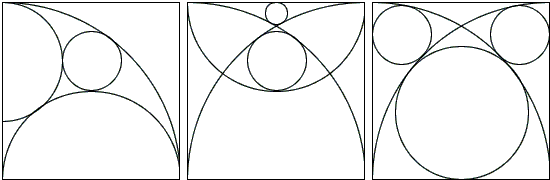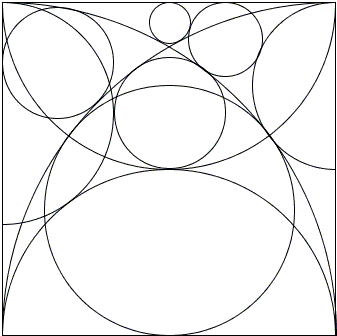## Sangaku and The Egyptian TriangleThree sangaku that require to determine the relative radii of the circles shown can be solved by a direct application of the Pythagorean theorem, see below, but if combined into a single (and a little amplified, sangaku-ready) configurationreveal existence of possible relationships not obvious when they are studied separately. The configuration in fact, as was noted by L. Bankoff and C. W. Trigg quarter of a century before sangaku grew in popularity after a 1998 Scientific American article, is rich with surprises, the main being the numerous sightings of the famous 3:4:5 triangle.The latter is most often referred to as the Rope-stretchers triangle and sometimes as the Egyptian triangle. (Both because of the belief that this simplest of the Pythagorean triangles was used by the ancient rope-stretchers in construction and, in particular, in construction of the Great Pyramids. Sometimes, however, the term Egyptian triangle is preserved for the one related to the dimensions of the pyramid of Cheops and the golden ratio.)For convenience, assume the side of the square is 24 so that DF = FC = 12. Also, for simplicity, a circle with center X say, will be denoted (X). AH is tangent to (F) in G. Thus also FG = 12. The radius FG extends to meet BC in J and is perpendicular to AH.

HC = HG as tangents to (F) from H. For the same reason AG = AD = 24. FH bisects ∠CFG and FA bisects its supplementary angle ∠DFG.

From here, ∠AFH = 90° and FG is the altitude to the hypotenuse of right ΔAFH. We, therefore, have FG2 = AG·GH, or 122 = 24·GH. Wherefrom GH = 6 and so HC = 6.

Then HB = 18, AH = 30, AK = 15, KG = 9, EK = 9, FK = 15, QK = 3, CJ = 16, FI = 8, EI = 16, AI = 20 (by the Pythagorean theorem), HJ = 10 ( ΔFGK is similar to ΔJGH), BJ = 8, GJ = 8, FJ = 20, IQ = 4.

We see that triangles FCJ, HGJ, FGK, AKE, AEI, AEM, and ABH are all 3:4:5 triangles.Let T be the intersection of AI extended with (A). Then AT = 24 and, since AI = 20, IT = 4 = IQ. Which says that the circle (I) of radius 4 is tangent to (A), (B), (F), and (E). If we extend AT further and let R on AT be on the vertical tangent SR to (I), then, for one, ΔIRS is similar to AEI and, hence, is also 3:4:5. Since IS = IQ = 4, IR = 20/3, SR = 16/3, RT = 8/3. If U is the intersection of SR and CD, then

 RU = FI - SR = 8 - 16/3 = 8/3 = RT.

In addition, if V is on BC and VC = RU, then VH = 10/3, RV = 8 and, by the Pythagorean theorem, HR = 26/3, so that again RX = 8/3. It follows that the circle (R) with radius 8/3 is tangent to (A), (I), (H) and CD. As an extra, it is also tangent to (W) with radius 3/2. ΔHVR is 5:12:13. The right triangle with vertical and horizontal legs and hypotenuse RW is 7:24:25.

### References

1. L. Bankoff, C. W. Trigg, The Ubiquitous 3:4:5 Triangle, Math Magazine, v 47, n 2 (Mar., 1974), pp. 61-70
2. H. Fukagawa, D. Pedoe, Japanese Temple Geometry Problems, The Charles Babbage Research Center, Winnipeg, 1989

Write to:

Charles Babbage Research Center
P.O. Box 272, St. Norbert Postal Station
Winnipeg, MB## Sangaku